# Maharashtra Board Practice Set 1 Class 7 Maths Solutions Chapter 1 Geometrical Constructions

Balbharti Maharashtra State Board Class 7 Maths Solutions covers the 7th Std Maths Practice Set 1 Answers Solutions Chapter 1 Geometrical Constructions.

## Geometrical Constructions Class 7 Practice Set 1 Answers Solutions Chapter 1

Question 1.
Draw line segments of the lengths given below and draw their perpendicular bisectors:
i. 5.3 cm
ii. 6.7 cm
iii. 3.8 cm
Solution:
i.Line AB is the perpendicular bisector of seg PQ.

ii.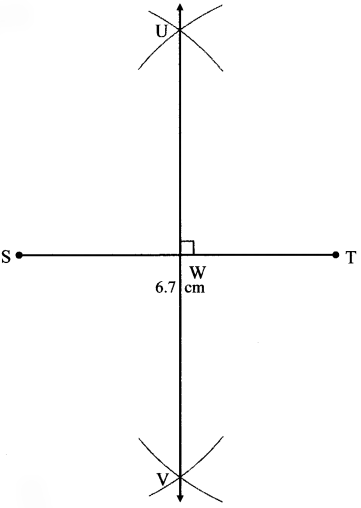Line UV is the perpendicular bisector of seg ST.

iii.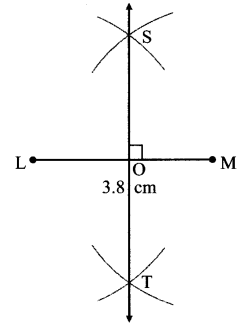Line ST is the perpendicular bisector of seg LM.

Question 2.
Draw angles of the measures given below and draw their bisectors:
i. 105°
ii. 55°
iii. 90°
Solution:
i. 105°ii. 55°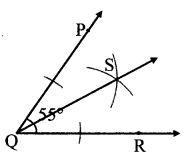iii. 90°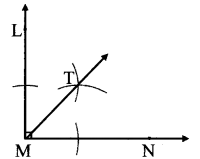Question 3.
Draw, an obtuse-angled triangle and a right-angled triangle. Find the points of concurrence of the angle bisectors of each triangle. Where do the points of concurrence lie?
Solution: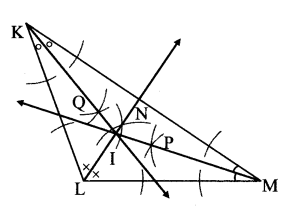The points of concurrence of the angle bisectors of both the triangles lie in the interior of the triangles.

Question 4.
Draw a right-angled triangle. Draw the perpendicular bisectors of its sides. Where does the point of concurrence lie?
Solution:The point of concurrence of the perpendicular bisectors of the sides of the right angled triangle lies on the hypotenuse.

Question 5.
Maithili, Shaila and Ajay live in three different places in the city. A toy shop is equidistant from the three houses. Which geometrical construction should be used to represent this? Explain your answer.
Solution:
Since, Maithili, Shaila and Ajay live in three different places, lines joining their houses will form a triangle.
The position of the toy shop which is equidistant from three houses can be found out by drawing the perpendicular bisector of the sides of the triangle joining the three houses.
The shop will be at the point of concurrence of the perpendicular bisectors.

Maharashtra Board Class 7 Maths Chapter 1 Geometrical Constructions Practice Set 1 Intext Questions and Activities

Question 1.
Draw a line segment PS of length 4cm and draw its perpendicular bisector. (Textbook pg. no. 1)

1. How will your verify that CD is the perpendicular bisector? m∠CMS = __°
2. Is l(PM) = l(SM)?

Solution: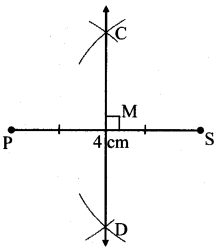1. Here, m∠CMS = 90°
2. Also, l(PM) = l(SM) = 2cm
∴ line CD is the perpendicular bisector of seg PS.